SBOS947A July   2019  – June 2020

PRODUCTION DATA.

1. Features
2. Applications
3. Description
1.     Device Images
4. Revision History
5. Pin Configuration and Functions
6. Specifications
7. Detailed Description
1. 7.1 Overview
2. 7.2 Functional Block Diagram
3. 7.3 Feature Description
4. 7.4 Device Functional Modes
8. Application and Implementation
1. 8.1 Application Information
2. 8.2 Typical Applications
9. Power Supply Recommendations
10. 10Layout
11. 11Device and Documentation Support
1. 11.1 Device Support
1. 11.1.1 Development Support
2. 11.2 Documentation Support
4. 11.4 Support Resources
6. 11.6 Electrostatic Discharge Caution
7. 11.7 Glossary
12. 12Mechanical, Packaging, and Orderable Information

• D|8
• DSG|8
• DSG|8

#### 8.2.1.2 Detailed Design Procedure

Designing a high-side current monitor circuit is straightforward providing the amplifier electrical characteristics are carefully consideration so that linear operation is maintained. Other additional considerations, such as the input voltage range of the analog to digital converter (ADC) that follows the current monitor stage, must be kept in mind while configuring the system.

Consider the design of a TLV2186 high-side current monitor with an output voltage range set to be compatible with the input of ADC with an input range of 3.3 V, such as one integrated in a microcontroller. The full-scale input range of such a converter is 0 V to 3.3 V. The TLV2186 can be operated from a single 24-V supply, referenced to ground. Although the TLV2186 is specified as a rail-to-rail input/output (RRIO) amplifier, the linear output operating range (like all amplifiers) does not quite extend all the way to the supply rails. This linear operating range must be taken into consideration.

The TLV2186 is powered by 24 V; therefore, the device is easily capable of providing the 3.3-V positive level, or even more if the ADC has a wider input range. However, because the TLV2186 output does not swing completely to 0 V, the specified lower swing limit must be observed in the design.

The best measure of an op amp linear output voltage range comes from the open-loop voltage gain (AOL) specification listed in the Electrical Characteristics table. The AOL test conditions specify a linear swing range 300 mV from each supply rail (RL = 10 kΩ). Therefore, the linear swing limit on the low end (VoMIN) is 300 mV, and 3.3 V is the VoMAX limit, thus yielding an 11:1 VoMAX to VoMIN ratio. This ratio proves important in determining the difference amplifier operating parameters.

An optimum load current, ILOAD of 10 A is used as an example. In most applications, however, the ability to monitor current levels well below 10 A is useful. This situation is where the 11:1 VoMAX to VoMIN ratio is crucial. If 11 A is set as the maximum current, this current must correspond to a 3.3-V output. Using the 11:1 ratio, the minimum current of 1 A corresponds to 300 mV.

Selection of the current sense resistor RS comes down to how much voltage drop can be tolerated at maximum current and the permissible power loss, or dissipation. A good compromise for a 10-A sense application is an RS of 10 mΩ. That value results in a power dissipation of 1 W, and a 0.1-V drop at 10 amps.

Next, determine the gain of the TLV2186 difference amplifier circuit. The maximum current of 11 A flowing through a 10-mΩ sense resistor results in 110 mV across the resistor. That voltage appears as a differential voltage, VR, that is applied across the TLV2186 difference amplifier circuit inputs:

Equation 1.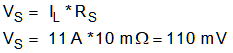The TLV2186 required voltage gain is determined from:

Equation 2.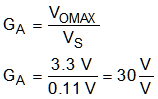Now, checking the VoMIN using IL = 1 A:

Equation 3.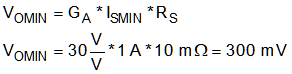The complete TLV2186 high-side current monitor is shown in Figure 45. The circuit is capable of monitoring a current range of < 1 A to 11 A, with a VCM very close to the 24-V supply voltage.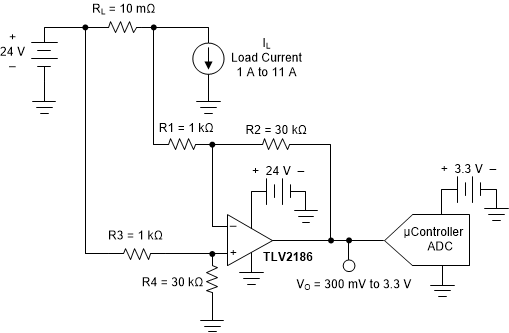Figure 45. TLV2186 Configured as a High-Side Current Monitor

In this example, the TLV2186 output voltage is intentionally limited to 3.3 V. However, because of the 24-V supply, the output voltage could be much higher to allow for a higher-voltage data converter with a higher dynamic range.

The circuit in Figure 45 was checked using the TINA Spice circuit simulation tool to verify the correct operation of the TLV2186 high-side current monitor. The simulation results are seen in Figure 46. The performance is exactly as expected. Upon careful inspection of the plots, one possible surprise is that VO continues towards zero as the sense current drops below 1 A, where VO is 300 mV and less.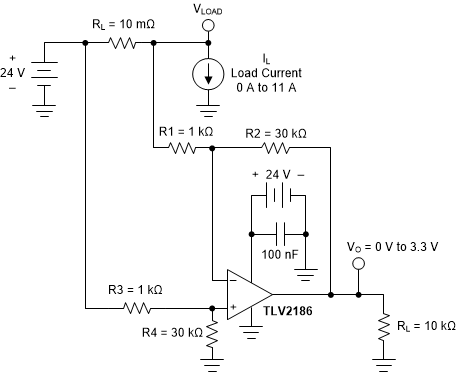Figure 46. TLV2186 High-Side Current-Monitor Simulation Schematic

The TLV2186 output, as well as other CMOS output amplifiers, often swing closer to 0 V than the linear output parameters suggest. The Electrical Characteristics table lists under the OUTPUT subsection VO, which is an output slam to the rail measure. It is not an indication of the linear output range, but instead how close the output can move towards the supply rail. In that region, the amplifier output approaches saturation, and the amplifier ceases to operate linearly. Thus, in the current-monitor application, the current-measurement capability may continue well below the 300 mV output level. However, keep in mind that the linearity errors are becoming large.

Lastly, some notes about maximizing the high-side current monitor performance:

• All resistor values are critical for accurate gain results. The resistor pairs of [R1 and R3] and [R2 and R4] must be matched as closely as possible to minimize common-mode mismatch error. Use a 0.1% tolerance, or better. Often, selecting two adjacent resistors on a reel provides close matching compared to random selection.
• Keep the closed-loop gain, GA, to which the TLV2186 difference amplifier is set, to a reasonable value. Doing so reduces gain error and can be used to maximize bandwidth. A GA of 30 V/V is used in the example.
• Although current monitoring is often used for monitoring dc supply currents, ac current can also be monitored. The –3-dB bandwidth, or upper cutoff frequency, of the circuit of is:

Equation 4.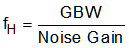where

• GBW is the amplifier unity gain bandwidth; 750 kHz for the TLV2186.
• Noise gain is equal to the gain as seen looking into the op amp noninverting input, as shown in Equation 5.
Equation 5.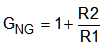For the TLV2186 circuit in Figure 45:

Make sure that the amplifier slew rate is sufficient to support the expected output voltage swing range and waveform. Also, if a single power supply such as 24 V is used, the ac power source applied to the sense input must have a positive dc component to keep the VCM above 0 V. The input voltage cannot drop below 0 V if normal operation is to be maintained.

The TLV2186 output can attain a 0 V output level if a small negative voltage is used to power the V– pin instead of ground. The LM7705 is a switched capacitor voltage inverter with a regulated, low-noise, –0.23-V fixed voltage output. Powering the TLV2186 V– pin at this level approximately matches the 300-mV linear output voltage swing lower limit, thus extending the output swing to 0 V, or very near 0 V. Doing so greatly improves the resolution at low sense current levels.

The LM7705 requires only about 78 μA of quiescent current, but be aware that the specified supply range is 3 V to 5.25 V. The 3.3-V or 5-V supply used by the ADC could be tapped as a power source.